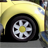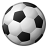# QlikView App Dev

Discussion Board for collaboration related to QlikView App Development.

Announcements
Now Live: Qlik Sense SaaS Simplified Authoring – Analytics Creation for Everyone: READ DETAILS
cancel
Showing results for
Did you mean:Not applicable

## Get value in Table A based on another Table B

Hello Friend,

I have two tables as follow.

Table A:

POINT FROMPOINT TOVALUE
02510
265020
517530
7610040

Table B:

CALCULATED POINTEXPECTED RESULT
55? (30)
20? (10)
74? (74)

Here Table A is master data.

I want to get expected result value in Table B based on Table A (Master Table) Point from and To.

for Ex.

Table B: Calculated Point = 55, Expected result = 30

Table B: Calculated Point = 20, Expected result = 10

Table B: Calculated Point = 74, Expected result = 30

Please let me know any solution.

Thanks

1 Solution

Accepted SolutionsCreator II

Hi Dipak

Create a Chart and use the following expression in that

=if((CALCULATEDPOINT>POINTFROM And CALCULATEDPOINT<POINTTO),VALUE)

Regards

Harsha

3 RepliesNot applicable
Author

Hi

as far as i remember  "interval match" function should solve the problem.

if not try "class"

best regards

chrisPartner - Creator

This is a script for IntervalMatchCreator II

Hi Dipak

Create a Chart and use the following expression in that

=if((CALCULATEDPOINT>POINTFROM And CALCULATEDPOINT<POINTTO),VALUE)